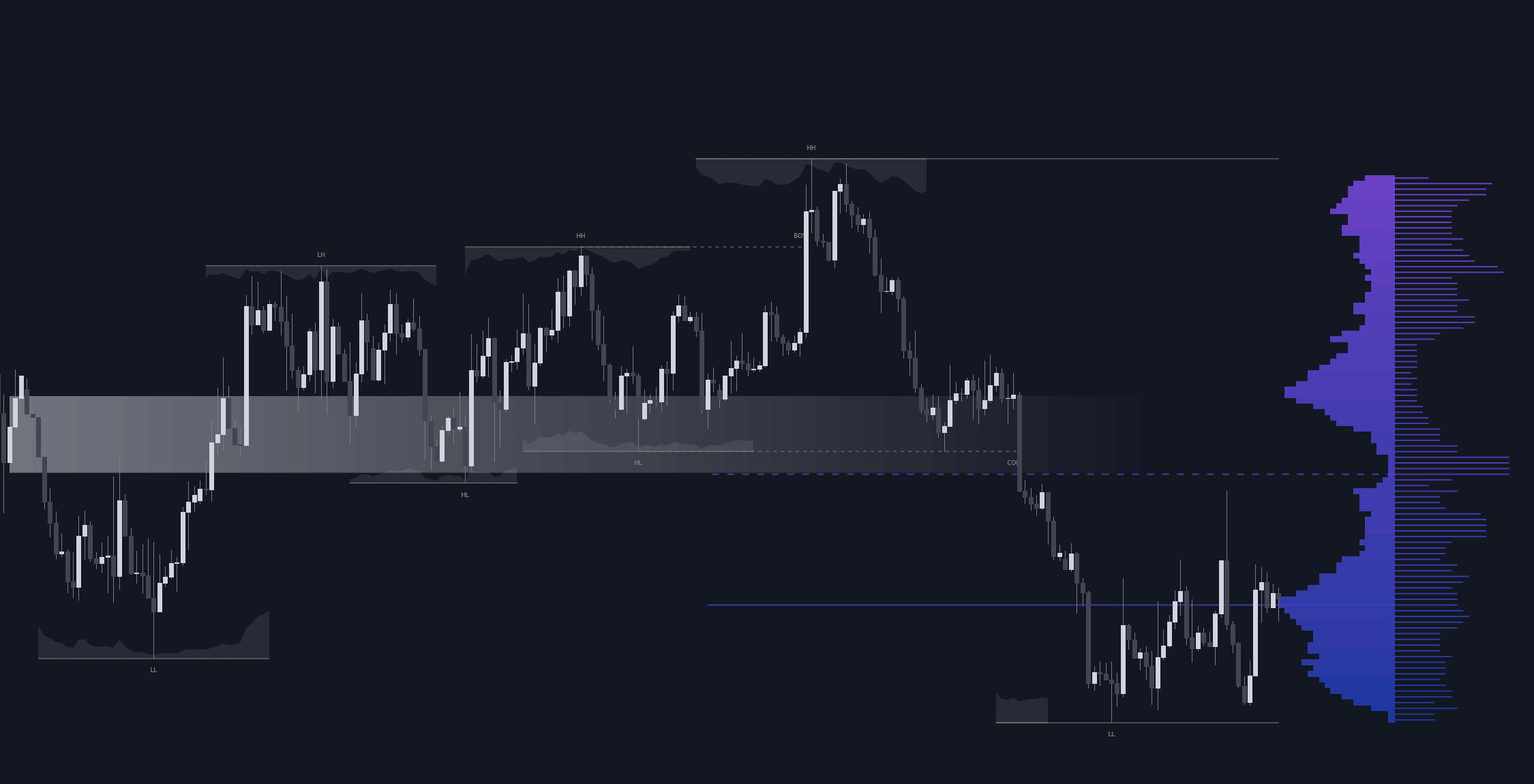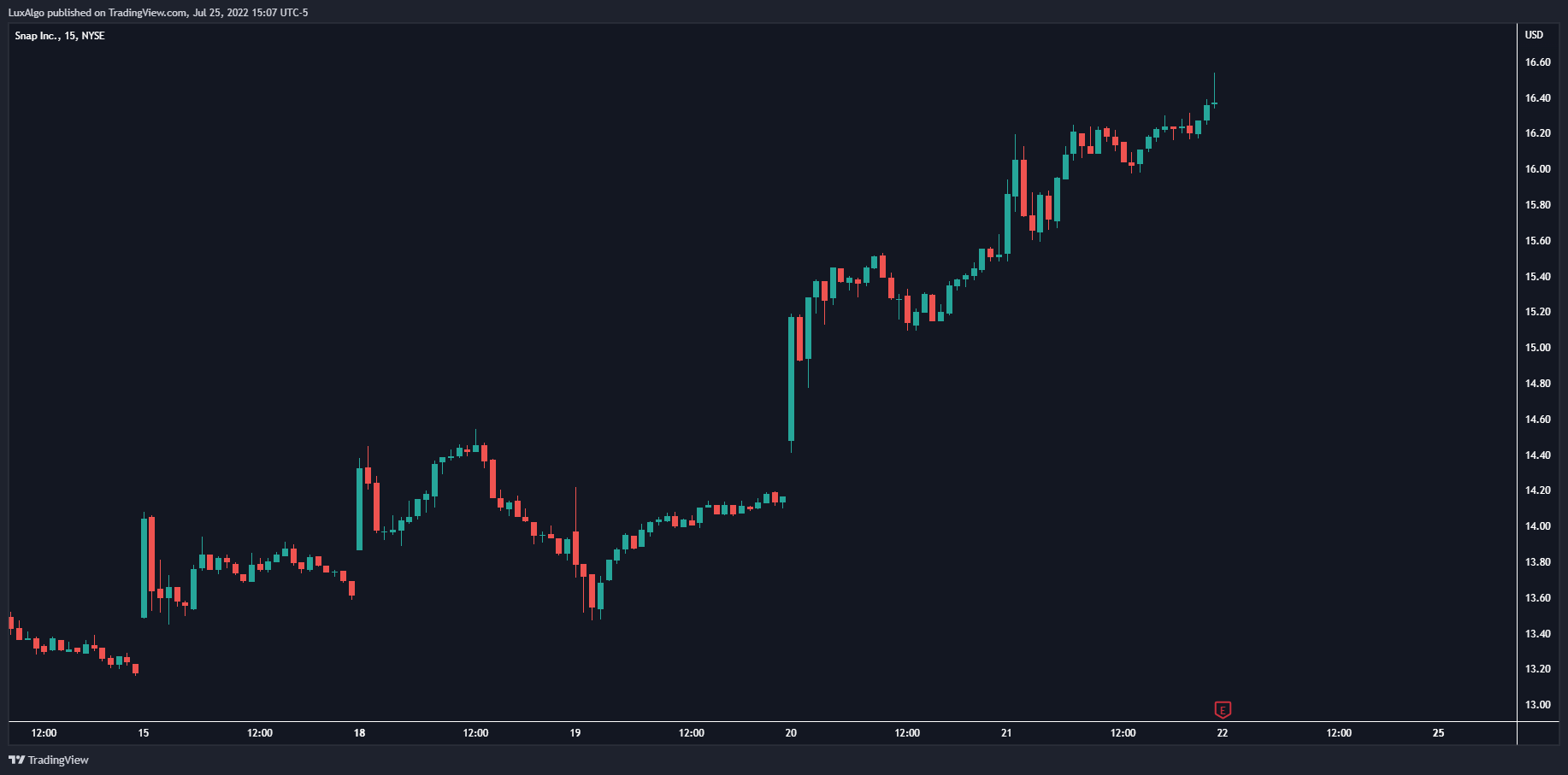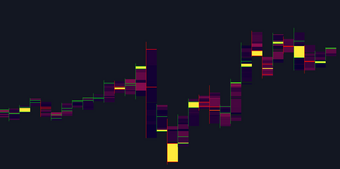# Linear Regression Histogram

This indicator is inspired by traditional statistical histograms. It will return the number of occurrences of price falling within each interval (bins) of the linear regression channel. This can be useful to highlight zones of interest within a trend.

This indicator is inspired by traditional statistical histograms. It will return the number of occurrences of price falling within each interval (bins) of the linear regression channel. This can be useful to highlight zones of interest within a trend.

#### Settings

• Length: Number of recent closing prices used for the computation of the linear regression .
• Bins Number: Number of intervals constructed from the linear regression channel.
• Mult: Multiplicative factor for the RMSE. Controls the width of the linear regression channel.
• Src: Input source of the indicator.

#### Usage

The indicator is constructed by dividing the linear regression channel range into a series of intervals (bins) of equal width. We then count the number of price values falling within each interval.

If a significant number of price values fall within a specific interval then that interval can highlight a potential zone of interest within a trend.

The zone of interest is highlighted in blue.

## Recent posts### Smart Money Concepts (SMC) Explained for Trading Forex, Stocks, & Crypto

##### September 9, 2022### The Best Time Frame for Trading (Actual Truth)

##### August 27, 2022### Liquidity Heatmap LTF

##### May 31, 2022